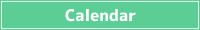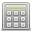<< < June 2023 > >>
SunMonTueWedThuFriSat
28 29 30 31 1 2 3
4 5 6 7 8 9 10
11 12 13 14 15 16 17
18 19 20 21 22 23 24
25 26 27 28 29 30 1## Percentage Decrease Calculator

Calculations such as how many are X percent decrease a certain number, and how many percent decrease a certain number are from the original number.

How much is X percent decrease a number?

% decrease for ?

What percentage is a number subtracted from the original number?

What % decrease is for ?

What is the original number when there is an X percent decrease?

When % decrease the original number is , what is the original number?

A percentage is a unit that expresses a percentage, and the whole is expressed as 100.

In the case of a percent decrease, the value obtained by subtracting the percentage of the decrease from the original amount of 100 will be the percentage after the decrease.

Original amount × (100 - Percentage of decrease) / 100 = Amount after percentage decrease

For example, if you have a class of 50 and the number of students is reduced by 20%, the number of students in the reduced class will be 50 × (100 - 20) / 100 = 40.

Decreased by 20 percent means that the original amount is 100 percent and it is now 80 percent. Because 80 / 100 = 0.8, it is the same as multiplying the original amount by 0.8.

## How to calculate percentage decrease

### How much is X percent decrease a number?

If you want to calculate the X percent decrease of a certain number, you can do it with
Some Number × (100 - Percent Decrease) / 100

Since the percentage represents the ratio when the original number is 100, by dividing the number obtained by subtracting the percentage of the reduction from 100 by 100, the ratio after the percentage decrease when the original number is 1 can ask.

You can calculate X percent decrease a number by multiplying the number by the percentage.

### What percentage is a number subtracted from the original number?

If you want to calculate what percentage of the original number a number decrease, you can do it with
100 - (Some Number / Original Number × 100)

A ratio is a value that represents the amount of something else relative to a source quantity, so you can find a ratio by dividing a number by the source number.

When expressed as a percentage, the whole is 100, so by multiplying that percentage by 100, you can calculate what percentage of the original number a certain number is.

If you want to calculate a percentage decrease, subtracting from 100 gives you the amount of decrease from the original number.

### What is the original number when there is an X percent decrease?

If you want to calculate some original number when you have a number with X percent decrease, you can calculate with
Some Number / (100 - Percent Decrease) × 100

Since it is a number that has X percent decrease from the original number, if you want to find the original number from a certain number, you can find it by dividing by the ratio.

The percentage is 100 of the total minus the percentage decrease.

Since it's a percentage, you can calculate the original number by multiplying it by 100.

###Simple Calculator

Save results in a temporary